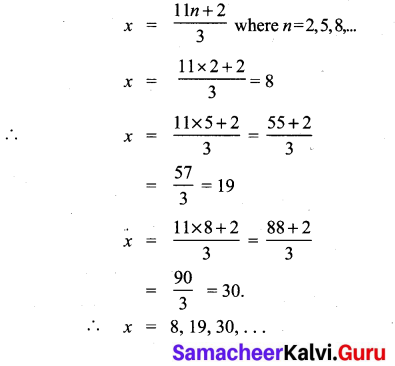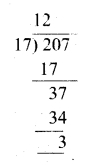## Tamilnadu Samacheer Kalvi 10th Maths Solutions Chapter 2 Numbers and Sequences Ex 2.3

10th Samacheer Maths Exercise 2.3 Solutions Question 1.
Find the least positive value of x such that
(i) 71 ≡ x (mod 8)
(ii) 78 + x ≡ 3 (mod 5)
(iii) 89 ≡ (x + 3) (mod 4)
(iv) 96 = $$\frac{x}{7}$$ (mod 5)
(v) 5x ≡ 4 (mod 6)
Solution:
To find the least value of x such that
(i) 71 ≡ x (mod 8)
71 ≡ 7 (mod 8)
∴ x = 7.[ ∵ 71 – 7 = 64 which is divisible by 8]

(ii) 78 + x ≡ 3 (mod 5)
⇒ 78 + x – 3 = 5n for some integer n.
75 + x = 5 n
75 + x is a multiple of 5.
75 + 5 = 80. 80 is a multiple of 5.
Therefore, the least value of x must be 5.

(iii) 89 ≡ (x + 3) (mod 4)
89 – (x + 3) = 4n for some integer n.
86 – x = 4 n
86 – x is a multiple of 4.
∴ The least value of x must be 2 then
86 – 2 = 84.
84 is a multiple of 4.
∴ x value must be 2.

(iv) 96 ≡ $$\frac{x}{7}$$ (mod 5)
96 – $$\frac{x}{7}$$ = 5n for some integer n.
$$\frac { 672-x }{ 7 }$$ = 5n
672 – x = 35n.
672 – x is a multiple of 35.
∴ The least value of x must be 7 i.e. 665 is a multiple of 35.

(v) 5x ≡ 4 (mod 6)
5x – 4 = 6M for some integer n.
5x = 6n + 4
x = $$\frac { 6n+4 }{ 5 }$$
When we put 1, 6, 11, … as n values in x = $$\frac { 6n+4 }{ 5 }$$ which is divisible by 5.
When n = 1, x = $$\frac { 10 }{ 5 }$$ = 2
When n = 6, x = $$\frac { 36+4 }{ 5 }$$ = $$\frac { 40 }{ 5 }$$ = 8 and so on.
∴ The solutions are 2, 8, 14…..
∴ Least value is 2.

10th Maths Exercise 2.3 Samacheer Kalvi Question 2.
If x is congruent to 13 modulo 17 then 7x – 3 is congruent to which number modulo 17?
Given x ≡ 13 (mod 17) ……(1)
7x – 3 ≡ a (mod 17) ……..(2)
From (1) we get
x- 13 = 17 n (n may be any integer)
x – 13 is a multiple of 17
∴ The least value of x = 30
From (2) we get
7(30) – 3 ≡ a(mod 17)
210 – 3 ≡ a(mod 17)
207 ≡ a (mod 17)
207 ≡ 3(mod 17)
∴ The value of a = 3

Ex 2.3 Class 10 Samacheer Question 3.
Solve 5x ≡ 4 (mod 6)
Solution:
5x ≡ 4 (mod 6)
5x – 4 = 6M for some integer n.
5x = 6n + 4
x = $$\frac{6 n+4}{5}$$ where n = 1, 6, 11,…..
∴ x = 2, 8, 14,…

10th Maths Exercise 2.3 In Tamil Question 4.
Solve 3x – 2 ≡ 0 (mod 11)
Solution:
3x – 2 ≡ 0 (mod 11)
3x – 2 = 11 n for some integer n.
3x = 11n + 2Samacheer Kalvi 10th Maths Question 5.
What is the time 100 hours after 7 a.m.?
Solution:
100 ≡ x (mod 12) (∵7 comes in every 12 hrs)
100 ≡ 4 (mod 12) (∵ Least value of x is 4)
∴ The time 100 hrs after 7 O’ clock is 7 + 4 = 11 O’ clock i.e. 11 a.m

10th Maths Exercise 2.3 Question 6.
What is time 15 hours before 11 p.m.?
15 ≡ x (mod 12)
15 ≡ 3 (mod 12)
The value of x must be 3.The time 15 hours before 11 o’clock is (11 – 3) 8 pm

10th Maths Samacheer Kalvi Question 7.
Today is Tuesday. My uncle will come after 45 days. In which day my uncle will be coming?
Solution:
No. of days in a week = 7 days.
45 ≡ x (mod 7)
45 – x = 7n
45 – x is a multiple of 7.
∴ Value of x must be 3.
∴ Three days after Tuesday is Friday. Uncle will come on Friday.

Exercise 2.3 Class 10 Maths Samacheer Question 8.
Prove that 2n + 6 × 9n is always divisible by 7 for any positive integer n.
9 = 2 (mod 7)
9n = 2n (mod 7) and 2n = 2n (mod 7)
2n + 6 × 9n = 2n (mod 7) + 6 [2n (mod 7)]
= 2n (mod 7) + 6 × 2n (mod 7)
7 × 2n (mod 7)
It is always divisible for any positive integer n

10th Samacheer Kalvi Maths Question 9.
Find the remainder when 281 is divided by 17.
Solution:
281 ≡ x (mod 17)
240 × 240 × 241 ≡ x (mod 17)
(24)10 × (24)10 × 21 ≡ x (mod 17)
(16)10 × (16)10 × 2 ≡ x(mod 17)
(165)2 × (165)2 × 2
(165) ≡ 16 (mod 17)
(165)2 ≡ 162 (mod 17)
(165)2 ≡ 256 (mod 17)
≡ 1 (mod 17) [∵ 255 is divisible by 17]
(165)2 × (165)2 × 2 ≡ 1 × 1 × 2 (mod 17)
∴ 281 ≡ 2(mod 17)
∴ x = 2

Maths Class 10 Samacheer Kalvi Question 10.
The duration of flight travel from Chennai to London through British Airlines is approximately 11 hours. The aeroplane begins its journey on Sunday at 23:30 hours. If the time at Chennai is four and a half hours ahead to that of London’s time, then find the time in London, when will the flight lands at London Airport.
Solution:
The duration of the flight from Chennai to London is 11 hours.
Starting time at Chennai is 23.30 hrs. = 11.30 p.m.
Travelling time = 11.00 hrs. = 22.30 hrs = 10.30 a.m.
Chennai is $$4 \frac{1}{2}$$ hrs ahead to London.
= 10.30 – 4.30 = 6.00
∴ At 6 a.m. on Monday the flight will reach at London Airport.